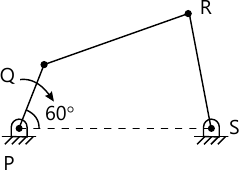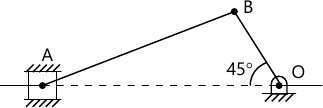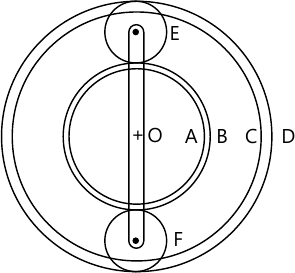MORE IN Kinematics of Machinery
VTU Mechanical Engineering (Semester 4)
Kinematics of Machinery
June 2015
Total marks: --
Total time: --
INSTRUCTIONS
(1) Assume appropriate data and state your reasons
(2) Marks are given to the right of every question
(3) Draw neat diagrams wherever necessary

1 (a) With a neat sketch define the following:
ii) Kinematic pair
iii) Kinematic chain
iv) Kinematic mechanism
v) Machine.
10 M
1 (b) Define inversion of a kinematic chain. With the help of a neat sketch explain inversions of single slider crank chain.
10 M

2 (a) With the help of a neat sketch, explain the working principle of crank and slotted lever mechanism.
10 M
2 (b) List various straight line generating mechanism. With the help of a neat sketch along with proof, explain how a Peaucellier mechanism generates a straight line.
10 M

3 PQRS is a four bar m/sm with link PS fixed as shown in Fig. Q3. The lengths of the links are; PQ=62.5 mm, OR=175 mm, RS=112.5mm, PS=200mm. The crank PQ rotates at 10 rad/s clockwise. Draw the velocity and acceleration diagrams when angles QPS=60° and Q and R lie on the same side of PS. Find angular velocity and angular acceleration of links QR and RS.20 M

4 (a) Locate all the instantaneous centres of the mechanism shown in Fig Q4(a). Determine the angular velocity of link AB and velocity of slider. Crank OB rotates CW at 150 rpm.

AB=  600 mm
OB= 150 mm10 M
4 (b) State Kennedy's theorem and state the properties of instantaneous centres (any two).
4 M
4 (c) With the help of a sketch, explain: i) Fixed instantaneous centre: ii) Permanent instantaneous centre and iii) Neither fixed nor permanent instantaneous centres.
6 M

5 The crank and connecting rod of a reciprocating engine are 300mm and 1500mm in length respectively. The crank rotates at 180 rpm in the clockwise direction. Determine the velocity and acceleration of the piston when the crank is 40° from IDC. Also determine the position of the crank for zero acceleration of the piston. Use complex algebra method.
20 M

6 (a) Compare involute and cycloidal tooth profiles of a gear.
4 M
6 (b) What is interference with respect to involute gears? State the methods to avoid interference.
6 M
6 (c) A pinion of 32 involute teeth and 4 mm module drives a rack. The pressure angle is 20°. The addendum of both pinion and rack is the same. Determine the maximum permissible value of addendum to avoid interference. Also find the number of pairs of teeth in contact.
10 M

7 (a) Sketch and explain: i) Compound gear train ii) Epicyclic gear train.
6 M
7 (b) In the epicyclic gear train shown in fig Q7(b) the compound wheels 'A' and 'B' as well as internal wheel 'C' and 'D' rotate independently about the axis '0'. The wheels 'E' and 'F' rotate on the pins fixed to arm 'a'. All the wheels are of the same module. The number of teeth on the wheels are TA=52, TB=56, TE=TF=36. Determine the speed of C if;
i) The wheel D fixed and arm 'a' rotates at 200 RPM in the clockwise.
ii) The wheel D rotates at 20 rpm counter clockwise and the arm 'a' rotates at 200rpm clockwise.14 M

8 (a) Sketch the following:
i) Disc cam with translating follower
ii) Wedge cam with translating follower.
iii) Cylindrical cam with oscillating follower.
6 M
8 (b) Draw the profile of a cam operating a knife edge follower having a lift of 30mm. The cam raises the follower with simple harmonic motion for 150° of the rotation followed by a period of dwell for 60°. The follower descends for the next 100° rotation of the cam with uniform velocity, again followed by a dwell period. The cam rotates at a uniform speed of 120 rpm and has a least radius of 20mm. What will be the maximum velocity and acceleration of the follower during the lift and the return?
14 M

More question papers from Kinematics of Machinery